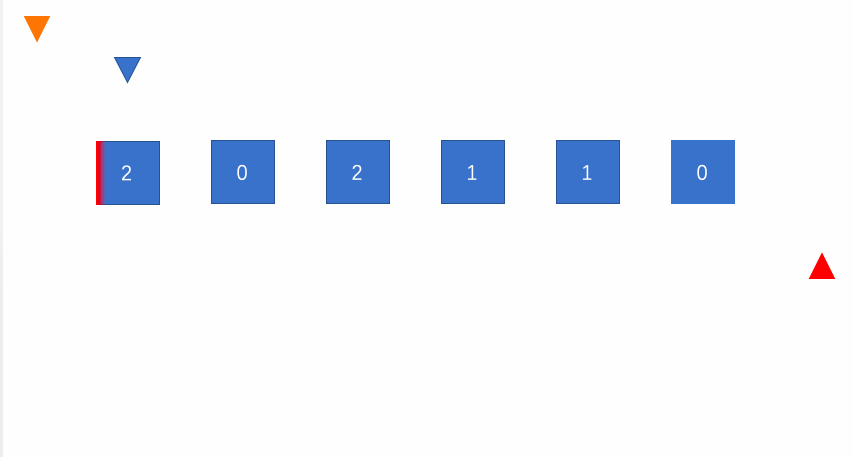# LeetCode 第 75 号问题：颜色分类

### 题目描述

``````输入: [2,0,2,1,1,0]

``````

• 一个直观的解决方案是使用计数排序的两趟扫描算法。
首先，迭代计算出0、1 和 2 元素的个数，然后按照0、1、2的排序，重写当前数组。
• 你能想出一个仅使用常数空间的一趟扫描算法吗？

### 题目解析

• 遍历`nums`，当`nums[i]`的值为1时，`i++`
• `nums[i]`的值为2时，`two`的值先减1，而后交换`nums[i]``nums[two]`，此时在观察`nums[i]`的值；
• `nums[i]`的值为0时，`zero++`，而后交换`nums[i]``nums[zero]``i++`;当 `i = two`时，结束循环。

### 动画描述### 代码实现

``````// 三路快速排序的思想
// 对整个数组只遍历了一遍
// 时间复杂度: O(n)
// 空间复杂度: O(1)
class Solution {
public:
void sortColors(vector<int> &nums) {

int zero = -1;          // [0...zero] == 0
int two = nums.size();  // [two...n-1] == 2
for(int i = 0 ; i < two ; ){
if(nums[i] == 1){
i ++;
}else if (nums[i] == 2){
two--;
swap( nums[i] , nums[two]);
}else{ // nums[i] == 0
zero++;
swap(nums[zero] , nums[i]);
i++;
}
}
}
};

``````